Edinburgh Online Graphics Dictionary

This dictionary section contains the terms that should be known by a person working with computer graphics. The terms were chosen to be specific to computer graphics, in the sense of image generation, rather than as used by human-computer interfaces or computation in general. We excluded terms that are either generic (e.g. color) or too uncommonly used.

These excellent resources can be consulted for further details about the terms defined here:

The following notation is used:

• point: p =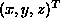• vector (i.e. a direction): d =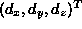• surface normal: n =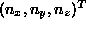• light source position: l
• viewer position: v
• camera position: c
• matrix: M
• rotation matrix: R

2D
Two-dimensional
3D
Three-dimensional
A-buffering
An antialiased extension to Z-buffering. An A-buffer identifies visible segments within a sub-pixel area which are represented with bit masks and area sampled for pixel intensity. The technique employs logical operations on the bit masks and thus avoids floating point geometry calculations.
Achromatic
Light without color. The quantity of light is the only attribute associated with achromatic light. In physical terms this is the intensity or luminance or in the psychological sense it is the perceived intensity in which case the term brightness is used. In the YIQ or YUV representations, this is the Y component. In the HSV representations, it is the V (value) component. In the HSL representations, it is the L (lightness or intensity) component.
An efficient way to evaluate parametric functions describing curves or surfaces. Each value of the function is determined as the sum of the previous value and a difference term. The distance between points at which the function is evaluated is adapted to the flatness of the function. The value can be a vector as well as a scalar, and this is useful for calculating B-splines.
Adaptive sampling is a method of reducing aliasing artifacts when rendering by adapting the sampling rate in response to the local characteristic of the object being rendered. This technique is often useful to reduce the jagged edges at the edges of objects (or jaggies).
A paradigm for representing data in a hierarchical manner by repeatedly dividing and classifying it until no further definition is necessary, given an error tolerance. E.g. see octree and quadtree.
In an additive color model, colors are defined as a sum of contributions from primary colors. The most commonly used additive color model is the Red-Green-Blue model.
Affine map/transform
A geometrical transformation from an affine space to another. In computer graphics it is used for 2D spaces (image) or 3D spaces (3D image). It is defined by : p' = M p, where M is a real matrix and p is a real vector. Affine transformations preserve parallelism.
Algebraic surface
A surface defined by the set of points for which an algebraic function is equal to a constant value. For an algebraic function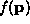at point p and a constant value c the surface S can be formally defined as: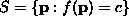Alpha blending/alpha-channel compositing
A technique for computing the color of a pixel when multiple structures contribute to the pixel ( e.g. at a region boundary where we want to avoid aliasing problems arising from partial pixel coverage or transparency). Alpha is the percent of a pixel covered by a given structure, and can be used as part of the color description of every pixel associated with a structure. Computing the resulting color of a combined pixel uses the alpha values of the source pixels, plus other information, such as any relation between the surfaces.
Alpha channel
The collection of alpha values associated with an image where each alpha value represents the coverage of each pixel in the image. The alpha values are used in the process of alpha blending.
Ambient lighting
A global (artificial) illumination level representing infinite diffuse reflections from all surfaces within a scene ensuring that all surfaces are visible (lit) particularly those without direct illumination. Ambient lighting is usually treated as a constant in local shading functions but is simulated directly in radiosity calculations.
Anaglyph
A stereoscopic picture consisting of two images of the same object, taken from slightly different angles, in two complementary colors. When viewed through colored spectacles, the images merge to produce a stereoscopic sensation.
Animation
(1) A medium that provides the illusion of a moving scene using a sequence of still images. (2) Techniques used in the production of animated films. In computer graphics this primarily concerns controlling the motion of computer models and the camera.
Anisotropic filtering
Image filtering that produces different amounts filtering (e.g. smoothing filtering in different directions at each pixel in an image. Two uses of anisotropic filtering in graphics are to: 1) produce textures with different spatial frequency distributions in different directions and 2) to reduce aliasing effects along edges without blurring the edges as much. Anisotropic filtering can be done in either the image or the frequency domains.
Antialiasing
Antialiasing is a method of reducing or preventing aliasing artifacts when rendering by using color information to simulate higher screen resolutions. In one technique, blurred pixels are introduced by filtering the image, or individual elements, to remove spatial frequencies that are greater than the pixel sample rate by convolution. If high frequencies remain they may cause other visual artifacts such as Moiré patterns. An alternative and often preferable technique is supersampling, where many samples per pixel are estimated and combined.
Artifacts/Artefact
A classifiable visual error. E.g., a loss of resolution when zooming into an image or incorrect depth sorting due to the painter's algorithm.
Atmosphere effects
Atmospheric effects arise because light is affected by the properties of the medium through which it passes. The main effects are attenuation, where distant objects get lower contrast (see depth cueing) and blurring, such as might occur with dust, fog or haze, which scatters the light.
Attenuation
1. Atmospheric attenuation: the simulation of the atmospheric attenuation from the object to the viewer which affects both the illumination strength and color. The attenuated illumination is computed by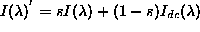where s is a scale factor ranging from 0 to 1,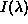is the illumination and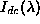is the depth-cue color.
2. Light source attenuation: a factor in the illumination equation used to simulate surface illumination depending on how far the surface is from the light source. It is defined by: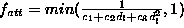where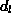is the distance between the source light and the surface, and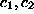and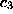are user defined constants associated with the light source.
Augmented reality
The idea that an observer's experience of an environment can be augmented with computer generated information. Usually this refers to a system in which computer graphics are overlaid onto a live video picture or projected onto a transparent screen as in a head-up display.
B-spline
A multi-segment spline curve representation based on local polynomials having continuity of curve orientation and curvature at the points (knots) where different segments join. Cubic b-splines are popular, but linear, quadratic, quartic, etc. splines are also used. The B in B-spline stands for basis, because the b-spline segments are formed from the weighted sum of four local basis functions. The local shape of the spline segment is controlled by four control points; in the case of b-splines, these control points do not lie on the curve itself ( i.e. b-splines are not interpolating). One important advantage of b-splines is that the movement of a control point affects only four segments of the curve. B-spline surfaces can be defined from b-spline curves lying in both directions on the surface. Here, 16 non-interpolated control points are needed per patch, but each patch then has tangent and curvature continuity where it joins a neighboring patch. The b-spline is defined over a uniform parameter domain, and is evaluated as a simple polynomial function. More complex forms, such as NURBS, relax these assumptions.
Backfacing polygons
Polygons whose surface normals point away from the camera position, which can be easily tested by the dot product of the polygon surface normal n and the ray v from the viewer to the polygon. A polygon is backfacing if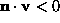. For closed objects there is no need to draw backfacing polygons as they are always occluded by non-backfacing polygons.
Background color
The intensity level of pixels which are not intersected by any of the displayed surfaces.
Backwards ray tracing
Backwards ray tracing is used to render a scene on a view plane by tracing imaginary "eye rays" from the viewer's eye to the surface of the objects in a scene, to determine the objects' visibility. A grid on the view plane is used to cast eye rays from the center of projection (the viewer's eye). It is convenient for the grid to correspond to the pixels of the display screen. For every pixel on the view plane, an eye ray is cast from the center of projection, through the center of the pixel and into the scene. The pixel's color is determined by the eye ray's point of first intersection with an object in the scene.

The basic backwards ray tracing algorithm can be extended to render shadows in a scene. This extension involves firing an additional ray from the first point of intersection to each of the scene's light sources. If the ray intersects with an object on its path to the light source, then the point of first intersection is in shadow for that light source. The combination of the effect of each ray to the light source determines the first intersection point's color.

Basis spline
A spline curve or surface that can be formulated as a weighted sum of polynomial basis functions. Commonly known as a B-Spline.
Beam tracing
Beam tracing is a method of rendering similar to ray-tracing but using an arbitrarily shaped projection, commonly a polygonal cone, rather than a single ray. It is an improvement on ray-tracing since it reduces the CPU overhead and reduces aliasing artifacts by taking advantage of known spatial coherence in the beam.
Bézier curve
A spline curve that (in the usual case of a cubic Bézier curve) is represented by four control points defining a cubic polynomial.
Bicubic surface
A type of parametric two variable polynomial surface patch where the polynomials are cubic in both parameters.
Bilinear filtering
An averaging technique applied to the color values of adjacent pixels so that textures look smooth rather than blocky. It aims to make the texture looks more realistic
Bilinear interpolation
An algorithm for interpolating image data in order to estimate the intensity or color of the image in between pixel centers. The interpolated value is calculated as a weighted sum of the neighboring pixel values.
Binary space partition tree
A method for representing a polyhedron that explicitly uses the planes that bound the polyhedron. The technique represents the object as a binary tree, with planes at each non-leaf node. The planes bound a face of the polyhedron, and divide space into two subregions, which in turn can be further bounded by the two children at a node. Leaf nodes (at the edge of the tree) are either completely object or completely free space. A similar idea can be used in 2D for representing polygons. This representation is useful for hidden surface removal and point classification (determining whether a point is inside or outside the object).
BitBlt/RasterOp
An abbreviation of bit block transfer. This is an efficient technique for copying rectangular arrays of pixels that exploits the fact that computer memory is organized into multi-bit words.
Bitmap
Strictly a one-bit-per-pixel representation for a defined area of a display.
Bits per pixel
The number of bits used to describe the color or intensity of a pixel. For example, using 8 bits for to store a value from the RGB color model would permit 3 bits to be used for both red and green values and 2 bits for the blue value. Blue gets a smaller range because the human eye contains less blue cones and is thus is less sensitive to blue variations.
Blend surface
A surface added to two or more others surfaces to provide a continuous join between them.
Blitter
A blitter is a special-purpose chip or hardware system used for fast implementations of bitmapped graphics. Blitters are used to copy sections of video memory from one place to another. During the copy operation several source areas may be used and logical operations may be performed on them. One application of blitters is the provision of fast animated graphics, known as sprites.
Boundary representation/B-rep
A paradigm for representing graphical data in terms of the boundaries of the objects involved. E.g., representing a cube as a collection of bounding faces, or a polygon by its edges.
Bounding box/volume
The smallest regular shaped box that encloses an object, usually rectangular in shape. Bounding boxes are used to accelerate tests such as visibility or ray-object intersection by providing a pre-test which can eliminate many cases.
Bresenham's algorithm
A technique developed in the framework of raster graphics for generating lines and circles. These algorithms use only integer arithmetic, avoid rounding and perform an iterative computation of the primitive points by approximating the distance to the nearest pixel center along either the x or y axis. These characteristics make for efficient algorithms.
Brightness
The perceived intensity of a radiating object.
Bump mapping
A technique used to increase the realism of a surface by changing how light reflects from that surface. Usually, the surface normal at a given point on a surface is used in the calculation of the brightness of the surface at that point. In bump mapping, the true surface normal n is perturbed a small amountn as a function of position on the surface. The perturbation can be regular, so as to give a regular textured shape to the surface, or it can be random, so as to increase the natural appearance of the surface. Part of what gives this techniques its appeal is that the original surface maintains its original (usually smooth) shape, and the bump-mapping distortion is specified by a compact function of shape. This is usually much simpler and more compact than specifying the surface texture by explicitly representing the textured surface.
Abbreviation of Computer Aided Design. In the context of graphics, CAD refers to the use of computer based models of objects for visualization or testing as an aid in the design process.
Camera
A virtual viewpoint in world space with position and view direction to provide a view of a scene in the same way as a photographer would position a camera.
Candela
Derived from candle and denoted by the symbol "cd", it is the basic SI unit of luminous intensity. It is defined as the radiation intensity, in a perpendicular direction, of a surface of 1/600000 square meter of a black body at the temperature of freezing platinum under a pressure of 101325 newtons per square meter.
Canvas
A two-dimensional region of graphics information. The canvas may be displayed on screen or be recorded in off-screen display memory.
Cartesian coordinates
A common system of representing a point in two or more dimensions using an ordered set corresponding to its projection on a spanning orthogonal base set. Commonly encountered Cartesian coordinate systems are the XY plane 2D coordinate system, (row,column) 2D image coordinate system and XYZ 3D scene coordinate system. 3D coordinates in graphics are usually specified with x and y being aligned with x and y on the screen, +x is to the right, +y is upwards, and +z goes into the space 'behind' the screen. This is a left-handed coordinate system with the property that most z-values are thereby positive. It's why z-buffers are called z-buffers when they are actually depth-value buffers.
CAVE
An immersive virtual environment where the viewer stands inside a room upon whose walls are projected images. The images may be in stereo requiring stereo shutter glasses to be worn. The name CAVE comes from Computer Augmented Virtual Environment.
Caustic
The effect given when light is transmitted through a specular surface and then strikes a diffuse surface. If the specular surface is of high curvature the light will be tend to be focused. When this effect is taken into account, rendered scenes involving liquids or glass are much more photorealistic. Caustics can also arise when light is reflected from a specular surface. The classic example is the caustic on the surface of a liquid. Refraction may make it happen too. The caustic shape is the envelope of the reflected rays.
Center of projection/viewpoint
Part of the model representing the the projection from a 3D space (the world) to 2D planar space (the image). It is the point of intersection of all the straight projection rays emanating from the object points in the 3D space and intersecting the projection plane to form the projection.
Chroma
1) A characterization of how much a color differs from both the pure color and the grey of the same intensity. Also called saturation. 2) The color component of a composite video signal.
Chromaticity coordinates/tristimulus coordinates
Chromaticity coordinates are based on the Commission Internationale de l'Éclairage (CIE) color scheme, which uses three standard (but physically unrealizable) primary colors called X, Y and Z. (These are different from red, green and blue, and are chosen to represent human color matching performance.) Any visible color c can be expressed as a weighted sum of these primary colors: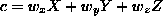. The weights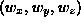are called the tristimulus values and are a way of objectively encoding all visible colors. (Actually, each set of weights represent an infinite set of colors which are indistinguishable.) Normalizing the colors by: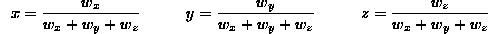generates the chromaticity coordinates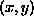, which are independent of the brightness of the color. Note that z = 1 - x - y, so we can recover z, but we have lost the absolute brightness of the color.

Chrominance
Information describing hue, or the color components orthogonal to the brightness. YUV and YIQ are chrominance/luminance color models.
Clipping
The selective removal of an object disjoint with the display area or the non-visible parts of an object that does intersect the display area. Parts of an object intersecting the display area may lie outside of the display area or be partially or fully obscured by another intersecting object.
Collision detection
Collision detection is used in a virtual environment to monitor the relative locations of solid objects. If the virtual environment manager detects that the proximity of two or more objects is sufficiently close, a collision event occurs. As a result of this event the object's movement can be controlled so their surfaces do not intersect. In an environment which models a natural system, the kinetic energy of a moving object is (partially) transferred to the object it collides with, making the second object move.
Color keying/chroma keying
Using the pixel color of one image to designate that pixel data from another image should replace the first pixel's color. The first image might be a binary image, which would select regions of interest from the second image. Another use is in blue-screening, where an actor works against a blue background. In the output image, the blue pixels get replaced by another image. For example, a weather map can be placed behind the weather presenter who is actually standing in front of a blue screen.
Color models
A color model is a method of specifying a color (position) in color space, often using a co-ordinate system. Examples include RGB and the Munsell Color System.
Color space
A mathematical space defining a range and encoding of colors. E.g. see RGB, LUV, HSV, HSL, YIQ, YUV and XYZ.
Compositing
The process of combining multiple images into a single image. Usually this is performed in films to make a computer graphics generated character appear on a previously filmed background. The term is also used in traditional photographic manipulation to refer to the process by which cel animation is recorded onto film under a rostrum camera. In film the 'mechanical' process is usually called matte photography (see color keying), and the process, when used in film sequences is ambiguously called traveling matte.
Concave/convex polygon
A concave polygon has the property that some points within its area can be joined by a line segment that passes outside the polygon. A convex polygon has the property that any line segment joining two points belonging to the polygon area is completely inside the polygon.
Cone tracing
An alternative to ray tracing in which cones are projected from the camera center through each pixel, where the intersection of the cone and the scene model is used to determine the pixel's color.
Contour
This is an image curve, often used to represent the set of points where a given function has a given constant value. A familiar example is a contour line on a topographic map. Here the contour denotes where the land has a given elevation. Another type of map contour might denote the boundary between increasing and decreasing population density. The equivalent concept in 3D is the level surface or isosurface.
Contrast
The range of colors in an image. Increasing the contrast of a color palette makes different colors easier to distinguish, while reducing the contrast makes them appear washed out.
Control point
One of a set of points which control the shape of a curve by their intuitively by position. The curves may go through some (see Bézier curve, an interpolating spline) or all (e.g. the Catmull-Rom interpolating splines) of the control points. Positioning is often interactive and the points are combined by blending functions to generate the shape desired. See also B-spline and Bézier curve. Note the distinction between knots and control points: in an interpolating spline, knots and control points are at the same positions in space. In a quadratic or higher order approximating spline they are in different places: the knots lie on the curve and control points lie near the knots, but not on the curve.
Convex hull
The convex hull of a given set of points is the smallest convex set that contains all the points.
Coons patch
A Coons patch is a form of parametric bicubic spline representation for surface patches. It allows explicit control of patch boundary position and tangent plane continuity. It is an example of a lofted surface.
Coordinate system
A coordinate system is a minimal set of mutually orthogonal vectors which span a given space. All points or vertices in the space may then be represented using a linear combination of these spanning vectors.
CSG/Constructive solid geometry
A paradigm for representing 3D shapes in terms of mathematically based compositions of geometric primitives. Any volumetric primitives can be used provided the primitive can satisfy an 'inside-outside' test which uniquely partitions points in the space near it. Typically, boolean set theoretic composition operators (e.g. intersection, union, difference) are used. Affine transformations may be applied to alter the shape of the primitives. For example, the exterior of an igloo may be represented as the union of a sphere and a cylinder, intersected with a cube.
Cuberille
A representation of 3D space consisting of a regular array of cubes, often referred to as voxels.
Data visualization
The set of techniques used to turn a set of data into visual insight. It aims to give the data a meaningful representation by exploiting the powerful discerning capabilities of the human eye. The data is displayed as 2D or 3D images using techniques such as colorization, 3D imaging, animation and spatial annotation to create an instant understanding from multi-variable data.
Delta frame
The difference between two consecutive images. Often used in video compression algorithms that exploit the temporal coherence of image sequences.
Depth buffer/Z-buffer
A method for solving the visible (or hidden) surface problem using two aligned pixel buffers or images. The first buffer stores the current color of the pixel and the second buffer stores the distance from the viewer to the surface. When rendering a point a on a scene surface, if the distance from the observer to a is greater than that of a previous point b that projects to the same image pixel, then point a can be ignored (as it cannot be seen). If the distance to a is less than the stored distance to b, then distance and color of a replace the color and distance buffer entry of b. A z-buffer is often efficiently implemented as a hardware buffer with entries aligned with pixels. Unfortunately, these z-buffers suffer a lot from aliasing effects and A-buffers are much better at dealing with visibility problems at sub-pixel accuracy.
Depth complexity
A measure of the complexity of an algorithm. It is equivalent to the number of pieces of data written to a framebuffer divided by the total number of pixels in the framebuffer, when a whole frame is rendered.
Depth cueing
Objects closer to the viewer appear brighter and more distinct than distant objects. Thus more distant objects or distant parts of objects are displayed with less intensity to simulate this phenomenon and enhance perception of depth.
Diffuse reflection
The portion of light that falls on a facet (small piece of the surface) which is radiated diffusely in all directions.
Direction cube
A technique used for representing spatial directions, often used by recursive direction decomposition algorithms. The cube is placed at the origin and aligned so that the coordinate axes are orthogonal to the faces. Each face of a cube is subdivided into a number of squares. Each square represents a collection of similar directions. Subdividing the squares on a face increases the resolution of the directions.
Directional lighting
A light source that radiates in such a way that rays from it are non-parallel.
Dissolve
An animation effect that is a transition between two sequences involving a fade from one directly to the other.
Dithering
One of many processes for reducing the total number of colors present in an image while retaining visual fidelity. Dithering can be done by interleaving pixels of selected colors to locally approximate the desired color. Dithering can be applied to either a color or a greyscale color space and may be necessary due to a limited number of colors available on the display device.
Double-buffering
A mechanism for duplicating the frame-buffer memory by using a two buffer system in which the image in one buffer is displayed while the image in the other buffer is computed. The newly created image is then displayed by swapping buffer pointers rather than having to copy memory. Double buffering allows the CPU to have uninterrupted access to one of the buffers while the video controller has access to the other.
Edge merging
The process of replacing the edge of a polygon with the adjacent edges of neighboring polygons to prevent cracks appearing during rendering.
Emittance
The light emitted by a surface. This may have different intensities and spectral characteristics in different directions.
Explicit surface
A surface representation in which the z coordinate is expressed as a function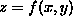of the x and y coordinates.
Extended light source
A light source with surface area which will cast shadows with both umbra and penumbra and thus is more difficult to model than a point source.
Face/facet/patch normal
A solid object can be constructed from many surface pieces which fit together. Each piece is called a face/facet/patch. Its normal is the direction from the surface of the object that is perpendicular to the piece's surface.
Facet/faceting
A facet is a small piece (usually a planar polygon) of a larger surface. Faceting is the technique used to construct a surface from multiple facets; triangulation is an example of faceting.
Fading is a method of switching between video sources, or images, using a black image as an intermediate. Fading without this intermediate is called a dissolve.
False coloring
See pseudo-color.
Field rendering
In interlaced video, a single image frame is sent as two fields - composed of even scanlines and odd scanlines. Field rendering refers to a method of rendering where fields are rendered separately in order to reduce motion artifacts.
Fill/flood fill
These are techniques for coloring areas bounded by line edges. The algorithms that fill interior-defined regions (the largest connected region of pixels whose values are the same as a given starting pixel) are called flood fill algorithms.
Filter
1) An optical device that selectively attenuates the intensity of light passing through it according to the light's properties. Common filters attenuate light according to either wavelength or polarization state. 2) An algorithm that selectively modifies the intensity or color of image data according to the image's properties. 3) An element (software or hardware) which takes in a stream of data and produces a stream of results, on average one output for each input.
Shading a polygonal patch with a single color and intensity. The shade chosen is a function of a variety of factors, such as light source position, viewer position and surface normal, according to the shading model used. A single shade is how the patch would appear if the surface is genuinely planar, rather than just being approximated by polygons, and if several viewing environment conditions hold (distant viewer and light source).
Fogging
The blending of a color, often light grey, with parts of an image such that the farther objects become increasingly obscured. (See Atmosphere effects.) In other words, the contrast between the fog color and objects in the image gets lower the deeper an object appears in the scene. Fogging may be used to provide a back-clipping plane where objects too distant to be seen clearly are removed to speed up the rendering of a scene.
FPS/Frames-per-second/Feet-per-second
1) A measure of the speed of an animation in terms of the number of complete, fully rendered images or frames which can be displayed in one second. 2) The same, except FPS refers to the number of feet (30.48 cm) of cinema film displayed in one second.
Fractal
A fractal has statistical self-similarity at all resolutions and is generated by an infinitely recursive process. In reality, those fractals generated by finite processes may exhibit no visible change in detail after some stage so are adequate approximations. So, for computer graphics we can extend the definition to include anything that has a substantial measure of exact or statistical self-similarity. This is illustrated by three stages of the construction of the von Koch snowflake below where each straight edge is repeatedly replaced by a copy of the entire figure. Fractals are useful for generating natural appearing shapes or textures, such as land and cloudscapes.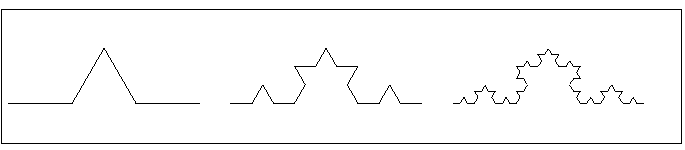Frame
A still two-dimensional image. Often a frame is a raster image as used in the frame buffer of a graphics display system. In computer animation frames per second is a measurement of the number of still frames displayed in one second to give the impression of a moving image.
Frame rate
The frame rate of a video source is determined by the speed at which it completes the rendering of a new image. This is limited by both the speed at which image data can be created and the rate at which video images can be presented on a display. For example the NTSC system redraws at 30Hz, PAL is 25Hz and computer displays are now usually 72-75Hz.
Frame size
A term used to refer to the dimensions of the array of pixels forming a frame of an animation, or alternatively the memory requirement and hence indirectly the resolution and dimensions.
Free-form/free-form surface
A surface that does not have a simple geometric description (e.g. not a plane or quadric surface). It is usually represented using a spline surface or a triangulated surface.
Frequency
The number of time that a periodic function or vibration repeats itself in a specified time or space. It is often measured in cycles per second, or cycles per centimeter, or cycles per degree of visual arc.
Fresnel equation
An equation used to determine the attenuation of unpolarized light reflected from a surface, given the refractive index of the surface material and the angle of incidence of the light relative to the surface normal.
Frustrum of vision
The visible region of 3D space. Projecting rays from the viewer through all pixels in the image plane defines an infinite pyramid-like solid shape within which all visible objects appear. The pyramid is then truncated by a distant plane to eliminate the space which is too far away to render, and by a closer plane which eliminates object too close to render. The space in between is the frustrum of vision.
Gamut
Normally refers to the full range of colors available in a color space. The gamut varies with resource: photographic film, printing inks, color displays, etc. A 24 bit color system has a gamut of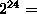16 million different colors. Moving between systems with different color gamuts will require quantization.
Gaze direction
View direction is specified as a target object rather than the more usual vector form from a camera or eye position and direction.
Generalized polygon
A generalized polygon is a planar shape that is constructed using an ordered number of vertices that are connected to form an enclosed polygonal area. It is a graphics engine's most abstract internal representation of a shape. Specific shapes such as square and triangle have a fixed number of vertices (3 and 4 in this example) and can be generalized using a generalized polygon. A generalized polygon may have holes or be concave.

Other shapes such as circle and ellipse have an infinite number of vertices. A generalized polygon can provide the graphics engine with an approximate representation by using a large number of vertices.

Gloss
An object is said to have a gloss surface when specular reflection is observed. This causes a highlight on the surface when a bright light is directed at the object.
Gouraud shading computes an intensity for each vertex of a polygon using Lambert-law shading and then interpolates the computed intensities across the polygon by performing a bilinear interpolation of the intensities down and then across scan lines. It thus eliminates the sharp changes at polygon boundaries.
Graphics application programmers interface (API)
A software library enabling a programmer to produce a graphical application, typically incorporating input handling (mouse, keyboard etc.). E.g. OpenGL, Java3D, Allegro.
Gray scale
A color space where colors are represented by their luminance values only, i.e. saturation and hue are zero.
Gupta-Sproull algorithm
A technique developed in the framework of Raster Graphics. It aims to reduce aliasing when doing line drawing.
Hedgehog
A visual representation of a surface in which the surface normals are rendered like pins sticking out of the surface.
Hidden line removal
A technique used in wireframe rendering (which is when one draws the straight line boundaries of the polygonal patches, or polyhedral solids that define the scene). If all boundaries are drawn, this is as if all surfaces and objects are transparent. If all surfaces and objects are opaque, then some boundaries would not be visible because they are hidden by closer surfaces. Removing the obscured or occluded portions of the boundaries is hidden line removal.
Hidden surface problem
Sometimes called visible-surface determination or hidden-surface removal. It is the problem of only displaying the parts of a surface in a scene which are visible to the user. For a scene to make sense to a user, any surface that is obscured by an opaque surface must not be rendered. For raster graphics, an example of a rendering algorithm which solves the hidden-surface problem is the z-buffer algorithm.
Highlight
The area of a glossy object over which specular reflection can be viewed. It is normally the color of the light source, not of the object.
Hollow fill
A three-dimensional object whose internal volume (defined as the space enclosed by the object's skin) is not rendered. Such three-dimensional solid objects are frequently used in virtual environments and are constructed using infinitely thin polygons to form the skin of the object. If the user's viewpoint is positioned within the skin of the object, the reverse of the surface will be rendered if there is sufficient illumination.
Homogeneous coordinates
Normally, the transformations for scaling, rotation and translation are treated differently. Scaling and rotation use matrix multiplication whereas translation uses vector addition. When the homogeneous coordinate system is used, all three transformations can be performed using matrix multiplication. This representation is commonly used in graphics systems because of its simplicity of representation and use. A homogeneous coordinate is expressed with an additional coordinate to the point. So, a two-dimensional point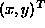is represented as a homogeneous coordinate by a triple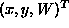. Two sets of homogeneous coordinates are equivalent if one is a multiple of the other. If the W coordinate is non-zero, we can divide each coordinate by W, transforminginto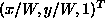; the numbers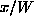and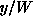are the Cartesian coordinates of the homogeneous point. If W is zero, the homogeneous coordinate is a point at infinity.
HSL/Hue-Saturation-Lightness
HSL, also known as HSI (Hue-Saturation-Intensity) is a color space used to represent images. HSL is based on polar coordinates, while the RGB color space is based on a three-dimensional Cartesian coordinate system. Intensity is the vertical axis of the polar system, hue is the relative angle and saturation is the planar distance from the axis. HSL is thought to be more intuitive to manipulate than RGB space. For example, in the HSI space, to change red to pink requires only changing the saturation parameter.
HSV/Hue-Saturation-Value
A color space that describes color using three basis components: hue, saturation and brightness. (See also HSL and Munsell color system.)
Hue
A perceptual term referring to the colorimetry quantity 'dominant wavelength' of a color. Hue can be used together with saturation and luminance to define the HSL color space.
Illuminance
The amount of light falling into a patch of unit surface area. It is measured in lux.
Illuminant
A source of illumination.
Image-based rendering
An approach to rendering in which objects and environments are modeled using image data instead of geometric primitives.
Image file format
A representation (usually binary) used by a computer system as an agreed format to store an image. Examples of image file formats include the Graphics Interchange Format (GIF) and Tagged Image File Format (TIFF).
Immersive VR/Virtual reality
A system where a user's field of view is completely filled by the display medium and the user can interact with the visualization in a natural way such as pointing, grabbing, head movement to change view, etc. The user is also shielded from external factors such that the overall perception is one of being immersed within the visualization.
Implicit surface
An implicit surface is defined using an implicit equation given by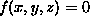for some function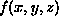. The equation restricts the interaction of x, y and z to ensure that the point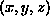is confined to the surface.
Inbetweening
Inbetweening is the generation of intermediate transition positions from a given start and end point or keyframes. This technique is often used in animation, where a lead artist generates the beginning and end keyframes of a sequence (typically 1 second apart), a breakdown artist does the breakdowns (typically 4 frames apart), and an `inbetweener' completes the rest.
Indexed 16 and 256 color images
An indexed color image consists of a set of references to values stored in a color table or palette. The palette, which is often contiguous in an image file, lists all the colors as sets of coordinates in color space. An indexed 16-color image contains a palette with 16 color entries (4 bits), whereas in an indexed 256 color image 256 colors are listed (8 bits).
Interlaced display
A technique for displaying images at a higher resolution than the monitor. Two images consisting of every second row of pixels are alternately displayed during every screen refresh (e.g. every fiftieth of a second). There is hence a flickering artifact.
Interpenetration
The surface of one object passing through the surface of another.
Interreflection/Mutual Illumination
A phenomenon that occurs when a surface reflects light from other surfaces in its environment. The effects range from more or less sharp specular reflections that change with the viewer's position to diffuse reflections that are insensitive to viewer's position.
A measure of the amount of light energy incident on a unit area of surface per unit time. Measured in Watts per square meter.
Isometric projection
This is a form of orthographic projection in which the direction of projection and surface normal of the image plane are parallel to one of these eight directions { (1,1,1), (1,1,-1), (1,-1,1), (1,-1,-1), (-1,1,1), (-1,1,-1), (-1,-1,1), (-1,-1,-1) }.
Isosurface
A technique used in three-dimensional data visualization where a surface is drawn around points in three-dimensional space that represent the same data value. For example, the set of points { (x,y,z) : f(x,y,z) = c } where c is a given constant. (See implicit surface.)
Iterated function system
A finite collection of affine mappings in the plane which are combinations of translations, scalings and rotations. Each mapping has a defined probability and should be contractive, that is, scalings are less than 1. Iterated function systems can be used for the generation of fractal objects and image compression.
Jittering
Jittering is performed by displacing sample locations that are initially spaced regularly. Typically, this involves randomly shifting uniformly positioned sample points horizontally and vertically. Such a sample point is usually in the center of the pixel which is perturbed to some other location within it. Jittering adds noise to the rendered image; the advantage of jittering is that the human eye tolerates noise more easily than it tolerates aliasing artifacts. Consequently, humans perceive the jittered image as being of a higher quality.
Keyframe
An image that is stored in some way to be used as a reference point. Key frames are often used in animation.
Knot
A knot is usually the join point between spline curve segments. (If the spline is a bundle of e.g. cubics model, then a knot is the place where one cubic stops and another starts.)
Lambert's law
A shading model in which the diffuse component of the brightness of a point on a surface is estimated as a scaled cosine of the angle between the surface normal and the direction from the point to a light source.
Level-of-detail blending
When rendering models which are defined with levels-of-detail, artifacts can occur when one level-of-detail is replaced with another. This is known as 'popping' and can be reduced by blending one level with the next when the transition takes place.
Leveling
A process applied to an image in order to have global uniform illumination. There are many techniques for leveling. The simplest consists in subtracting from the original image the image of the background taken under the same conditions and then expanding the contrast of the difference.
Light source
Line clipping
Selecting the portion of a line segment that lies inside of a clipping window. If the line intersects the window boundary, then the line is split into two or three segments, one of which moves with the clipping window and the other(s) remain unmoved.
Line drawing
An image created only from points connected by lines. It can be described using a series of end-point coordinate information,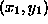and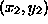, for each connecting line. This can be combined with a weight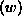which denotes the thickness of the connecting line.
Linear depth cuing
Linear depth cueing is a rendering technique used to give the effect of depth to an image. This is achieved by intensities in the image plane being reduced linearly with respect to the distance from the plane. (See Attenuation.)
Local light source
Light source that directly (i.e., not by reflection or transmission) illuminates a point on a surface.
Luminance
The absolute quantity of radiation emitted from a given light source.
Luminosity
The relative quantity of radiation emitted by a light source.
LUV space
A color space similar to the XYZ model, except that the components are scaled to be perceptually linear. The human eye perceives brightness on a logarithmic scale, and hence LUV components are logs of the corresponding XYZ values.
See Lambert's law.
Metaball
Metaball modeling is based on the production of objects using spheres that attract and cling to each other according to their proximity to one another and their field of influence (the size of their attractive field). This form of modeling may also use cubes and other shapes, depending upon the modeler. Metaball modeling is particularly useful for creating organic objects and animation effects such as a group of mercury balls moving together and combining to form an object like a soda can.
Metameric match
Two colors that appear to be the same to a human. They may not have identical spectral distributions, but, because humans measure light using only three cone types, the differences are indistinguishable.
Microfacets
An approximation used in developing an improved specular reflection component to surface shading. The Torrance-Cook (or Cook-Torrance) physical surface shading model assumes that a surface is composed of a set of tiny planar patches, each placed according to a distribution that depends on the surface. The microfacet model leads to a reflection function that gives more realistic values for the direction and intensity of the specular component of surface reflection.
MIP mapping
MIP mapping is a technique of precomputing anti-aliased texture bitmaps at different scales, where each image in the map is one quarter of the size of the previous one. When the texture is viewed from different distances, the correct scale texture is selected by the renderer so that fewer rendering artifacts are experienced, such as Moiré patterns.

MIP is apparently an acronym relating to the latin `multum in parvo', meaning many things in a small place - since the texture contains the same content at different scales. A MIP mapped texture requires 4/3 times the storage of the original (1 + 1/4 + 1/16 + ...).

Moiré pattern
A watered appearance usually provided as texture on the surface of objects. It arises from the interference between two overlapping patterns with a similar spatial frequency.
Monochromatic
Light (or other source of electromagnetic radiation) having only one wavelength.
Morphing
A continuous deformation from one keyframe or 3D model to another. In 3D this is often achieved by approximating a surface with a triangular mesh that can then be continuously deformed. In 2D, it is generally performed by either distortion or deformation.
Munsell color system
The Munsell color-order system is a way of precisely specifying colors and showing the relationships between colors. In this system, the color space has three parameters : hue, value and chroma (saturation). Munsell uses scales with visually uniform steps for each of these parameters. A Munsell Book of Color displays a collection of colored chips arranged according to these scales. The parameters are written in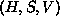form, known as the Munsell notation. (See HSV.)
Nit
An equivalent name for the unit of luminance: candelas per square meter.
NURBS
Non-Uniform Rational B-Splines. A class of piecewise parametric curves or surfaces where each curve segment or surface patch is described by a ratio of Non-Uniform B-Spline polynomials. B-splines are a class of polynomials whose coefficients depends on a set of control points. For the Uniform B-Splines each curve segment or surface patch is defined by a parameter domain of fixed length or area respectively, whereas in the Non-Uniform B-Splines the parameter domain does not have to be uniform. The Non-Uniform characteristic allows different levels of continuity between the curve segments and the surface patches whereas it is restricted to p-1 levels for the uniform case, where p is the degree of the polynomial. Thus, Non-Uniform B-Splines can interpolate points more accurately. Furthermore, rational forms can represent conic curves and are invariant under rotation, scaling, translation and perspective transformations. NURBS provide a superset of commonly used surfaces and has been adopted as the IGES (Initial Graphics Exchange Specification) for free-form surfaces.
Occlusion
Visual obstruction. An occlusion occurs when an opaque surface prevents another surface from being seen. When rendering, it is necessary to determine which surfaces are not occluded, a problem known as the hidden surface problem.
Octree
A space-occupancy representation used for representing 3D volumetric objects. It is a hierarchical representation, designed to use less memory than representing every voxel of the object explicitly. Octrees are based on subdividing the full voxel space containing the represented object into 8 octants by planes perpendicular to the three coordinate axes. Octants that completely contain a single object are denoted as being pure. Octants that contain multiple objects are recursively split into 8 new smaller octants. This splitting continues until all volumes are either pure, or some volume size limit is reached. A tree data structure can be used to represent the octree. Normally, the octree data structure will have only about as many nodes as there are voxels on the object surface, which can be much less than the total number of voxels in the object. Hence, an octree representation can save a lot of space when representing an object or scene.
One-sided surface
A surface rendered in such a way that only one side is visible. That side is usually facing the same direction as the surface normal.
Opaque
Impervious to light. An opaque surface will reflect light to some degree dependent on surface attributes. See also Specular reflection and Diffuse reflection.
Orthographic projection
A type of parallel projection where the direction of projection is the same as the surface normal to the projection plane. Specialist types of orthographic projection include front-elevation, top-elevation and side-elevation where the projection plane is perpendicular to the principle axis. Such projections, called isometric projection, are often used in engineering drawings as they preserve distances and angles.
Overlay
An image compositing method where an image is displayed over a background image.
Painter's algorithm
An algorithm for hidden surface removal, where objects are assigned priorities based on proximity to the camera position. When the image is rendered to the buffer the objects with higher priority overwrite those with lower priority. Although intuitive and simple to implement, this algorithm has been superseded by z-buffering.
Palette
The set of colors that may be used to compose an image.
Pan
Camera rotation about an axis (vertically) perpendicular to the camera's view direction.
Parametric
An approach to shape representation in which a curve or a surface is defined by a set of equations expressed in terms of a set of independent variables (i.e. the parameters). This representation is convenient for curvature and bounds computation and the control of position and tangency.
Parametric surface
A surface defined explicitly by the range of values of a parametric function. For a parametric function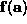that depends upon the parameter vector, the surface S can be defined formally as: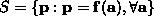Particle system
A technique for modeling irregular natural structures by a collection of independent objects, often represented as single points. Objects that have been represented using this technique include fire, smoke, clouds, fog, explosions, grass, etc. Each particle will have its own motion and property parameters, usually drawn randomly from a distribution (perhaps constrained by or linked to other particles, or other scene objects, such as grass being constrained to grow from a specified surface). Because natural effects based on particle systems need many particles for realistic appearance, rendering of particle systems often requires special-purpose methods that exploit the properties of the particular particle system.
Path tracing
Path tracing is an improvement on general ray-tracing techniques. Normal ray-tracing uses a constant factor to estimate the contribution of ambient light at a given surface point but path-tracing estimates the global illumination using, for example, Monte Carlo techniques. Images are thus generated using many paths through each pixel. Note that a degree of oversampling is always necessary, so this technique is computationally expensive.
Penumbra
That part of a shadow due to a light source which receives partial illumination from the source. By definition the source will be an extended light source and the penumbra always surrounds the umbra.
Perspective projection
Perspective projection is the complete projection model of a scene onto an image plane via a pinhole camera model. The perspective projection of any set of parallel lines which are not parallel to the projection plane converge to a vanishing point. In 3D, the parallel lines meet only at infinity and there is an infinity of vanishing points, one for each of the infinity of directions in which a line can be oriented.
A shading model for surfaces based on the interpolation of local surface normals at the vertices of a triangular patch. The technique is used for more realistic rendering of glossy surfaces.
Photo-realistic rendering
The process of rendering images so that they closely resemble a photograph. Such renderings must take into account reflective properties, light sources, illumination, shadows, transparency and mutual illumination.
Photometry
Making measurements from images. One example is creating a 3D scene description using stereo image analysis, and measuring the volume of an object in the model.
Pixel
A single discrete sample point of an image. Image size and resolution are defined in terms of number of pixels.
Pixel depth
The number of bits used to generate a color at each pixel. The number of different colors that can be displayed is equal to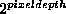. For instance a pixel depth equal to one means that only black and white colors could be displayed; with a pixel depth equal to four, sixteen different colors could be displayed.
Plenoptic function
The plenoptic function is the 5-dimensional function representing the intensity or color of the light observed from every position and direction in 3-dimensional space.
Point light source
A mathematically defined infinitely small point l from which light radiates. The point might be at infinity, in which all light rays are parallel, or it might be closer to the object, in which case light rays radiate outward in all directions. The amount of light radiated in different directions need not be uniform.
Point sampling
Point sampling algorithms are those which only solve for visibility at a finite number of discrete points. A typical example is ray tracing. They are generally used in simple renderers. There are four point sampling algorithms in common use today: z-buffering, painter's algorithm, ray tracing and the scanline algorithm. Other point sampling algorithms are generally variations on these. They have advantages over continuous algorithms because they are easier to understand and implement, faster and can generate a greater range of optical effects. It is difficult to generate photorealistic fully anti-aliased images using a point-sampling algorithm.
Polygon
A plane figure which is a closed contour of straight lines. A basic primitive in the graphical representation of objects.
Polygon fill
A series of ordered planar vertices connected to form an enclosed area. This area is then completely rendered using a specified color or texture.
Polyhedron
A 3D solid that is bounded by a set of polygons whose edges are each a member of an even number of polygons.
Polyline
A continuous line formed from one or more connected line segments. Polylines are specified by the endpoints of each segment.
Portals
A method for reducing framebuffer overdraw where visible areas of a 3D model are clipped before they are rendered. Small areas in the model are grouped as sectors and portals are the transition planes between them. An initial view frustrum is defined to be as large as the image plane. Then all visible polygons are clipped to this volume. A sector is rendered only if its portal is within the clipping frame. Then a new smaller frustrum is defined at that position and polygons visible from it are clipped and so on, recursively.
Procedural surface
A procedural surface uses external parameters supplied to a model that determines how the surface will be generated. For example, a procedural surface that generates a polygonal representation of a sphere at a specified detail is procedural; the actual surface is generated by the specified sphere diameter and the number of polygons that will make up the surface. An advantage of using this approach is efficient storage and replication since individual polygons need not be explicitly specified.
Procedural texture
A texture generated by a model controlled by external parameters.
Pseudocolor
Pseudocoloring recolors pixels with colored values as a function of the grey level value in the original monochrome image. Pseudocoloring is used because of the limitation of the human visual system to distinguish all the brightness range values.
Purity
The degree to which a color is saturated.
A curved surface defined by the equation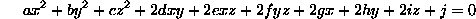Special cases of the surface include spheres, cones, cylinders, ellipsoids, hyperboloids, etc. The translation, rotation and scaling of a quadric surface is easy, as is the calculation of its surface normal, intersection with a ray and calculation of the z-value (given the x and y values).

A tree structure used to encode two-dimensional spaces, such as images. The image is recursively subdivided into subquadrants. At each subdivision the subquadrants are assigned a ``full'', a ``partially full'' or an ``empty'' label depending on how much the quadrant intersects the region of the interest in the image. The subdivision of partially full subquadrants continues recursively until all the subquadrants are homogeneous (full or empty) or a predetermined cutoff depth has been reached. The edges of the tree represent the different subquadrants and the nodes to which they point represent the subquadrant labels.
Quantization
1) The subsetting of data or a resource to enable or speed up processing. An example of the former is where a device has no more than an 8 bit color capability thus requiring a 24 bit image to be requantized to 8 bit color for processing. Subsetting large data sets can also speed up processing. An example of resource quantization is where the processing of a screenful of data in an image-based algorithm can be made much more efficient by subdividing the screen, perhaps on a binary basis, and applying the algorithm to smaller sections of the data. 2) Converting a continuous quantity into series of discrete values. For example, continuous images can be quantized into discrete pixels, color spaces can be quantized into a set of discrete colors, or continuous time can be quantized into discrete steps.
A measure of the amount of electromagnetic radiation leaving a point on the surface. More precisely, it is the rate at which light energy is emitted in a particular direction per unit of projected surface area. The projected surface area is the projection of the surface onto the plane perpendicular to the direction of radiation. It is found by multiplying the surface area by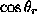, where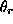is the angle of the radiated light to the surface normal.
A image rendering algorithm that allows diffuse and mutual illumination effects by evaluating the radiation of light from light sources and reradiation amongst surfaces. Radiosity calculations determine the steady state in the radiative transport of light around a closed volume. Essentially, the illumination leaving a patch is a proportion of the light reaching the patch from all the other visible patches in the closed volume. Patch surface normals are typically distributed everywhere and some patches are occluded or partly obscured from each other. The accumulation of these radiation-attenuating effects is summed up as the form-factor between each pair of patches. The main and most time-consuming part of the radiosity calculation is the calculation of these form factors.
Raster coordinates
Raster coordinates are an artifact of the method of CRT image reconstruction where pixels are addressed and illuminated in a top-to-bottom, left-to-right fashion. Hence, raster coordinates are the 2D coordinates of the current drawing position either in the image window or the hardware frame buffer.
Ray tracing
A rendering paradigm that aims to produce realistic images (rather than real-time) given a 3D model. The color of a pixel is determined by calculating the path of a ray of light passing through a point in the 3D model corresponding to the pixel. The path is traced back to a light source.
Recursive decomposition
An algorithm where space is divided into successively smaller pieces until a threshold is found. These algorithms can be used to draw curves by approximating them by a chain of line segments. This can also be used to render surfaces by subdivision algorithms, such as the hierarchical B-spline refinement algorithm.
Reflectance
Reflectance is a measure of the ability of a surface to reflect electromagnetic radiation ( e.g. light). It is equal to the ratio of the reflected flux to the incident flux.
Refraction
The phenomenon of a beam of light bending as the light's velocity changes. This occurs when the refractive index of the material through which the light is passing changes. Let i be the normalized incident ray vector (pointing towards the surface), which has unit surface normal n. If t is the transmitted (refracted) vector inside a transparent medium, then: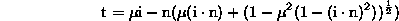where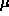is the ratio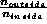of the refractive indices of the inside and outside media. (See Snell's law.)

Render
To create an image from a description of a scene, its objects and light sources and the viewer.
Resolution
This indicates the number of pixels per image. It is often represented in this format: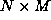where N and M are the number of pixels per column and row respectively.
Retroreflector
A type of surface with unusual reflectance characteristics, namely that it reflects light mainly back in the direction from which it came. This makes retroreflecting surfaces appear much brighter than matte surfaces, if the light source is in the same direction as the viewer, and dark otherwise. Retroreflecting surfaces are often found on road markings and signs.
RGB color model
The RGB (``red'', ``green'', ``blue'') color model describes a color as a positive combination of three appropriately defined red, green and blue primaries. If the r, g and b components are defined as scalars constrained to a value between 0 (no intensity) and 1 (maximum intensity) all the definable colors will be bounded by a cube and it is typical to describe RGB combinations as co-ordinates on the cube (r, g, b). For example pure red is (1, 0, 0) and the secondary color cyan is (0, 1, 1); darker colors have values closer to (0, 0, 0) (black) and lighter colors have values closer to (1, 1, 1) (pure white).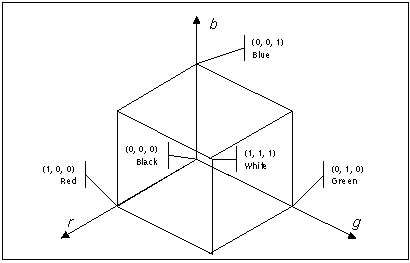RGB true color
An RGB color system with 24 bits per pixel color resolution. This gives a choice of over 16 million colors per pixel. Such a system is generally known as a true color or full color system.
Rotation
A rotation is a geometric transformation that changes the orientation of an object, extended light source or viewpoint. Specific rotations are often represented by a matrix R, which then transform point p to the new position Rp. Rotation and many other simple transformations can be done simultaneously if positions and directions are represented in homogeneous coordinates.
Saturation
A perceptual term referring to the colorimetry quantity 'excitation purity' of a color. Hue can be used together with saturation and luminance to define a HSL color space.
Scalar
A quantity which has magnitude but no direction.
Scaling
The process in which the size of an image or geometric representation is modified by multiplying each component of the representation's coordinates by constant factors. Scaling and many other simple transformations can be done simultaneously if positions and directions are represented in homogeneous coordinates.
Scanline algorithm
An algorithm that renders an image one row at a time, e.g. generates the image values for pixels left-to-right as it scans across the image. After one row is generated, the algorithm proceeds to the next row. One advantage of this algorithm is it can use less memory to generate the results for only single rows at a time. Another potential advantage is a reduction in computation as the set of object primitives that need to be rendered at each pixel along a scan line may not change very often, so some results calculated at one pixel can be used at the next.
Screen door transparency
A technique for rendering the transparency of an object. The key idea is to only render some of the pixels associated with the object, depending on how transparent the object is.
Sculptured surface
A highly flexible surface generated by the combination of surface patches which have both their boundary curves and interior blending functions defined by polynomials of, usually, at least order three. See also B-spline and Bézier curve.
Self-occlusion
A surface is self-occluding when: a) Light cast from behind the surface does not illuminate it. b) The light source is in front of the surface but some closer portion of the surface blocks the incoming light. c) The light source is in front of the surface and the surface is illuminated, but some closer portion of the surface blocks the light coming from the surface.
Coloring a surface according to its incident light. The color depends on the position, orientation and attributes of both the surface and the sources of the illumination. (See also Lambert's law, Phong shading and smooth shading).
A shadow map is a pre-computed array used to test whether points on object surfaces are in shadow. The array contains depth values from the viewpoint of a point light source giving the distance to the first object surface encountered. If a given pixel in the environment is not contained in the array then it is in shadow. This method is useful for quickly re-rendering an image from several different viewpoints or when several light sources are used - each would then have its own shadow map.
Skeleton
A framework capturing the structure of an object or shape constructed from a series of points connected by thin lines. In a similar way to a wireframe representation, a skeleton is used to increase the performance of the rendering system since it is not necessary to render solid surfaces. Objects represented using a skeleton can be given a skin by specifying a diameter from the skeleton used to render the surface. In cartoon animation, a skeleton is literally a line structure representing the position of the limbs of a figure and is not necessarily oriented along the medial axis.
A method of polygon shading where calculations are performed for the vertices and values for pixels inside the polygon are derived from linear interpolation of the vertex values.
Snell's law
A law defining how light is bent or refracted when it passes through a boundary between two dielectric media of different indices of refraction, such as air and glass or air and water. It is expressed by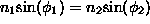where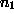and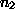are the index of refraction of the two media.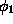and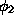are the angles which the boundary surface normal makes with the incident light ray and the refracted light ray respectively.
The process of orienting and moving through a virtual environment.
Spatial partitioning
A technique used to divide a large task into a series of smaller ones. The basic approach is to devise a pre-processing stage which determines spatially coherent groups for processing. This strategy is particularly appropriate for parallel architectures where the groups can be sent to different processing units.
Specular reflection
One component of light reflection at a surface point (see also diffuse reflection). Specular reflection is observed on ``shiny'' surfaces and is characterized by highlights on the surface. The amount and direction of specular reflection depend on the directions of the incident light and the viewing direction with respect to the surface normal.
Spline curve
A spline curve is defined using a set of control points (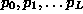). Every control point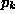has an associated blending function,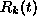, which is described within each span (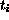,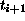). The blending function is a continuous piecewise polynomial, continuous at each knot and weighted by the polynomials. This gives a curve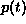, which is the union of the piecewise polynomials where all segments meet.
Staircasing
Lines are scan-converted to fixed pixel grid points. The illuminated pixels often do not lie on the true path of the line. The result is that displayed lines are normally jagged in appearance, an effect commonly known as the jaggies or staircasing. The effect can be reduced or eliminated by antialiasing.
The unit of solid angle. The solid angle corresponding to all of space being subtended is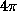steradians. Solid angle is defined as the surface area of a unit sphere which is subtended by a given object.
Stereo
The use of two images to generate a 3D description. E.g. two slightly different images are displayed in a each eye of a virtual reality head mounted display in order to induce an impression of 3D. Stereo matching is a process by which two images of the same scene are compared in order to deduce 3D information.
Stochastic sampling
A method of reducing the visual effects of aliasing by sampling in an irregular manner, rather than on a regular grid. Recognizable aliasing artifacts are replaced by noise, which viewers find less objectionable. (See also jittering.)
Superconic
Generalization of conic curve in which the trigonometric terms in the formula of the curve are raised to an arbitrary power to control the smoothness of the curve. It can be expressed by: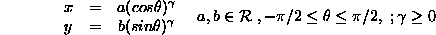A class of parametric surfaces, derived from the class of quadric surfaces, in which the trigonometric terms of the quadric equation, written in parametric form, are raised to a power. The exponents are known as the squareness parameters and are used to pinch or square off parts of the original quadric shape. A special case are the superconics.
Surface normal
Any surface that is smooth enough for at least one derivative calculation at a given point has a surface normal. This is a unit vector n that is perpendicular to the plane tangent to the surface at the given point. It is usually taken to be pointing outward away from the surface. Smooth surfaces have surface normals at every point. Planar surfaces have the same surface normal at every point that is not at the edge of the surface. At crease or fold edges, the surface normal is undefined.
Surface patch
This term has several usages in the graphics community: 1) a small piece of surface with arbitrary shape and size surrounding a surface point with a given surface normal or 2) a primitive element of a geometric surface description, such as a spline or triangulation patch. Graphics techniques that use the different surface patch representations are mainly related to surface representation, visibility analysis, illumination and reflectance.
Sweeping
The definition of a new object in a higher dimension produced by arbitrary movement of the originating object along a path in the space of the higher dimension. For example, one can create a cylindrical surface by sweeping a line about another line which is parallel.
Tesselation
A technique to construct a surface by a small set of figures which fit together. They are drawn repeatedly over the entire plane leaving no gaps.
Texel
The fundamental element of a texture map.
Texture map
A bitmap used to texture a 3D polygon model, including adjustments for perspective correction, where vertices of the object model are mapped onto the 2D texture bitmap. In addition to color and brightness, textures may also be encoded with the properties of transparency and specular reflectivity. This kind of texture may also be procedural in nature.

A possible side-effect of texture mapping occurs unless the renderer can apply texture maps with correct perspective. Perspective-corrected texture mapping involves an algorithm that translates texels, or pixels from the bitmap texture image, into display pixels in accordance with the spatial orientation of the surface.

A shading model that incorporates an ambient lighting component, a diffuse component (see Lambert's law) and a specular component.
Translation
Point M can be moved, or translated, to a new location M' by adding a vector T. More concisely : M' = M + T. Translation and many other simple transformations can be done simultaneously if positions and directions are represented in homogeneous coordinates.
Translucent
A characteristic of a material allowing light to pass through partially or diffusely.
Transparency
The ratio of the amount of light passing through a material to the amount of light incident on the material.
Triangulation
The transformation of a model into a mesh of triangles to facilitate speedy rendering or other computational geometry algorithms. The initial model might be a planar graph, free-form surface, polygonal model, point cloud data or volumetric data.
Trilinear filtering
A level of detail blending technique used in MIP texture mapping. Pixels are taken from multiple MIP maps and blended to produce the final color. The purpose is to remove the bands between adjacent pixels taken from different MIP maps.
Umbra
The part of the shadow created by an extended light source that is entirely cut-off from the source. It is surrounded by the penumbra that receives some light from the light source.
Vanishing point
A point in a perspective projection where parallel lines not parallel to the projection plane converge. A finite 2D projection of a point at infinity in 3D.
Vector
A list of numbers, typically Cartesian coordinates or a direction in 2D or 3D. E.g.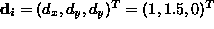.
Vector graphics
The earliest computer graphics displays were drawn on so-called vector displays, because the electron beam which produced the image was under software control. The beam followed a chain of vectors (i.e. a polyline) from one point to another. Vector graphics is sometimes referred to as line-drawing graphics
Vertex
The points in a model at which edges terminate. E.g. the eight corners of a cube, or the three corners of a triangle. Polyhedrons, polygonal surfaces and triangulations are composed of vertices, edges and faces.
Vertex normal
The direction vector pointing directly out of a polygonal/polyhedral model at a given vertex. This may be defined as the average of the surface normals of the faces adjacent to the vertex.
Viewpoint
The location of a virtual camera in a model.
Virtual camera
A set of parameters defining a 2D view of a 3D model. These might include: camera location, direction, camera twist - defining the upwards direction in the rendered image.
Virtual environments
An artificial environment maintained by a computer which a user may interact with or view.
Virtual reality
A simulation of a virtual environment which according to some must have an 'immersive' quality encouraging the feeling of being present in the environment. Technology used with virtual reality includes stereo image helmets, 360 degree screens but may be as simple as a standard monitor display.
Visible surface determination
During rendering of 3D scenes it is necessary to determine which objects occlude others in order that the scene looks correct, and time wastage may be prevented by not drawing shapes that will be overdrawn. Techniques include: culling backfacing polygons, Z-buffering and Warnock's algorithm.
Volume rendering
The visualization of 3D volume data. E.g. data sets such as MRI scans consisting of a volume of density samples or voxels.
Voxel
Volume element. A single datum in 3D volume data.
VRML
Virtual Reality Modeling Language. A 3D model description format suited to transfer on the WWW.
Warnock's algorithm
A spatial partitioning technique for depth sorting a list of polygons so that they may be rendered correctly. The algorithm subdivides the screen rectangle until it may be painted entirely in the color of the foremost polygon or the background color.
Warping
The manipulation of 2D images by arbitrary geometric (i.e. position) transformations of the pixels of some or all of an image. Some simple types of warping are stretching, scaling, rotating, skewing, shearing or perspective transform (perspective projection). This may be used to draw texture maps. Many simple transformations can be done simultaneously if positions and directions are represented in homogeneous coordinates.
Weiler-Atherton algorithm
A technique for clipping one generalized polygon with the boundary of another.
Wireframe
A minimal vector graphic rendering style in which only the edges of shapes are drawn. This is appropriate for polygonal objects, although many other surface representations may be quickly converted for faster rendering, typically for editing purposes.
XYZ color space
XYZ color space. A color model in which X specifies the red component and Y the green component. The blue component is 1-X-Y (the color components are scaled so that R+G+B=1). Z specifies the brightness.
YIQ color space
A chrominance/luminance color space model used in the American NTSC television standard, Y specifies luminance, I and Q specify chrominance. I specifies the red-orange/cyan (or blue-green) component, and Q specifies the green/magenta (or purple) component.
YUV color palette
A chrominance/luminance color space model used in the British PAL television standard, Y specifies luminance, U and V specify chrominance. U specifies the blue/yellow component, and U specifies the red/cyan (or blue-green) component. YUV is also called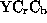.
Z-buffering
A technique for speeding up depth sorting (See visible surface determination) while rendering. As each primitive in the frustrum is drawn, the distance from the viewpoint is recorded in the Z-buffer or depth buffer. If a pixel has already been drawn with a closer Z value the new pixel value is not recorded.
Zoom pyramid
A data structure which stores an image at multiple size/resolutions. The zoom pyramid for a 640x320 image would include versions with sizes 240x160, 160x80, 80x40, etc. Zooming in to the image quickly is therefore possible.
Zooming
Viewing an image at different sizes. Zooming in creates an enlarged view of a portion of the scene in the image frame. Zooming out does the reverse.
Date of last change: Sept 12, 1999
Editor: Robert Fisher
Contributors: Anthony Ashbrook, Bob Fisher, Josh Hale, Eric McKenzie, Jon Meddes, John Patterson, Craig Robertson, Gordon Watson, Naoufel Werghi

There have been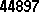accesses since January 2000.